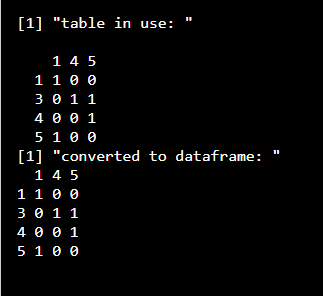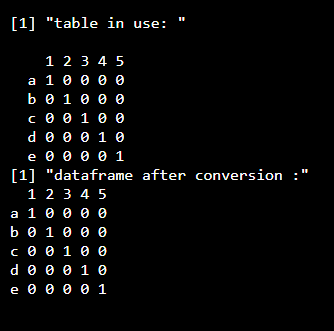How to convert table to dataframe in R?

• Last Updated : 08 Oct, 2021

In this article, we will discuss how to convert a given table to a dataframe in the R programming language.

Functions Used

• as.data.frame.matrix() will be taking the table as its parameter and will return the dataframe back to the user.

Syntax: as.data.frame.matrix(x)

Parameter:

• x: name of the table which is to be converted into dataframe

Returns: It will return the dataframe which is converted from the given data.

• table() function is used for the cross-classifying factors to build a contingency table of the counts at each combination of factor levels of the provided data in R language.

Syntax: table(…,exclude = if (useNA == “no”) c(NA, NaN),useNA = c(“no”, “ifany”, “always”),dnn = list.names(…), deparse.level = 1)

Returns:

This function will be returning the frequency table of the provided data back to the user.

Thus, we will first create a contingency table using table() function from a dataframe and then convert this table to a dataframe.

Given below are some implementations for this.

Example 1:

R

 data_gfg=data.frame(x=c(1,3,5,3,4),                    y=c(1,5,1,4,5)) table_gfg=table(data_gfg\$x,data_gfg\$y)print("table in use: ")table_gfg new_data_gfg=as.data.frame.matrix(table_gfg)print("converted to dataframe: ")new_data_gfg

Output:Example 2:

R

 data_gfg=data.frame(x=c("a","b","c","d","e"),                    y=c("1","2","3","4","5")) table_gfg=table(data_gfg\$x,data_gfg\$y)print("table in use: ")table_gfg new_data_gfg=as.data.frame.matrix(table_gfg)print("dataframe after conversion :")new_data_gfg

Output:My Personal Notes arrow_drop_up